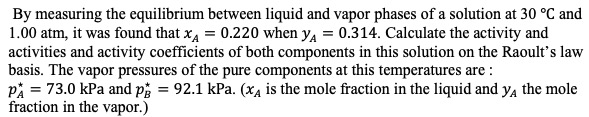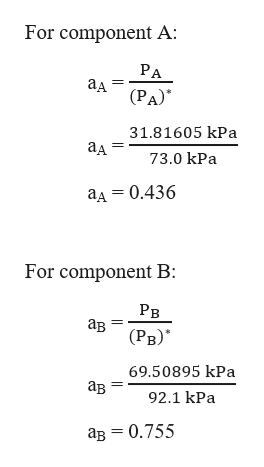# By measuring the equilibrium between liquid and vapor phases of a solution at 30 °C and1.00 atm, it was found that xA 0.220 when y 0.3 14. Calculate the activity andactivities and activity coefficients of both components in this solution on the Raoult's lawbasis. The vapor pressures of the pure components at this temperatures arep 73.0 kPa and p 92.1 kPa. (x4 is the mole fraction in the liquid and ya the molefraction in the vapor.)

Question
4 viewshelp_outlineImage TranscriptioncloseBy measuring the equilibrium between liquid and vapor phases of a solution at 30 °C and 1.00 atm, it was found that xA 0.220 when y 0.3 14. Calculate the activity and activities and activity coefficients of both components in this solution on the Raoult's law basis. The vapor pressures of the pure components at this temperatures are p 73.0 kPa and p 92.1 kPa. (x4 is the mole fraction in the liquid and ya the mole fraction in the vapor.) fullscreen
check_circle

Step 1

Given,

Total pressure, PT = 1.00 atm = 101.325 kPa

Mole fraction in liquid phase, xA = 0.220

Mole fraction in vapour phase, yA = 0.314

Vapour pressure of pure component A, PA* = 73.0 kPa

Vapour pressure of pure component B, PB* = 92.1 kPa

Step 2

Firstly, we need to find the partial pressures (PA and PB) of components A and B as:

Step 3

The activities (a) of the compone...help_outlineImage TranscriptioncloseFor component A: РА ад (РА)" 31.81605 kPа ад 73.0 kPa ад — 0.436 For component B: Рв ав (Рв)" 69.50895 kPa ав 92.1 kPa ав — 0.755 fullscreen

### Want to see the full answer?

See Solution

#### Want to see this answer and more?

Solutions are written by subject experts who are available 24/7. Questions are typically answered within 1 hour.*

See Solution
*Response times may vary by subject and question.
Tagged in

### Solutions